#### 期刊菜单

Hopfield Neural Network Based Approach to Recognizing the Verification Code

Abstract: In order to avoid malicious cracking of passwords, ticket brushing, forum water injection, hacking, etc., verification codes are born. It uses the method of forcing human-computer interaction to resist machine automation attacks. But accurately identifying the verification code is a difficult problem that has not yet been completely resolved. The research object of this article is a four-digit verification code. The method of segmentation analysis and Hopfield neural network is used to analyze the verification code recognition and its accuracy. Establish and train the Hopfield network to perform pattern recognition on the transformed vector of the captcha image. The conclusion of the comparison shows that the Hopfield neural network algorithm can get better test results and has the value of popularization and application.

1. 引言

2. 图像的预处理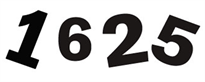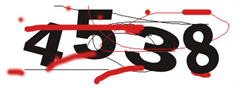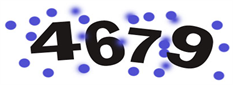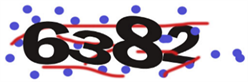Figure 1. Digital verification code

2.1. 灰度化

$g\left(i,j\right)=\text{Max}\left(R\left(i,j\right),G\left(i,j\right),B\left(i,j\right)\right)\text{​}\text{​}\text{​}\text{​}\text{​}\text{​}\text{​}\text{​}\text{​}\text{​}\text{​}\text{​}\text{​}\text{​}\text{​}\text{​}\text{​}\text{​}\text{​}\text{​}\text{​}\text{​}\text{​}\text{​}\text{​}\text{​}\text{​}\text{​}\text{​}\text{​}\text{​}\text{​}\text{​}\text{​}\text{​}\text{​}\text{​}\text{​}\text{​}\text{​}\text{​}\text{​}\text{​}\text{​}$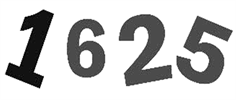(a)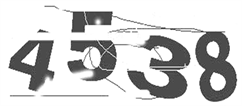(b)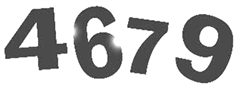(c)(d)

Figure 2. Retain the grayscale results of the maximum brightness method

2.2. 二值化

$g\left(i,j\right)=\left\{\begin{array}{cc}1& f\left(i,j\right)>T\\ 0& f\left(i,j\right)\le T\end{array}$

$\lambda =\frac{{\sigma }_{\beta }^{2}}{{\sigma }_{W}^{2}},\text{\hspace{0.17em}}K=\frac{{\sigma }_{T}^{2}}{{\sigma }_{W}^{2}},\text{\hspace{0.17em}}\eta =\frac{{\sigma }_{\beta }^{2}}{{\sigma }_{T}^{2}}$

${\sigma }^{2}\left(T\right)={W}_{A}{\left(\mu a-\mu \right)}^{2}+{W}_{b}{\left(\mu b-\mu \right)}^{2}$Figure 3. Maximum interclass method binarization

2.3. 中值滤波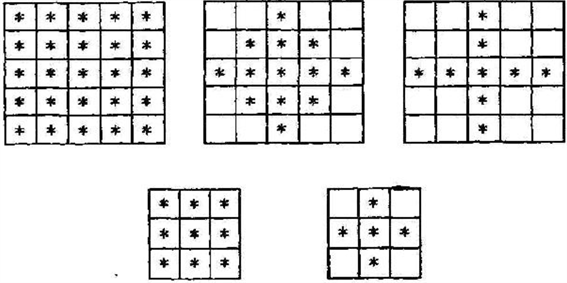Figure 4. Gray value recognition windowFigure 5. Median filter

2.4. 字符串分割和归一化

2.4.1. 边缘检测分割法

2.4.2. 归一化Figure 6. Image after edge detection

3. Hopfield网络识别模型的建立与验证

3.1. 神经网络的基本原理

Hopfield网络全连接网络 。图7就很好地展示出了一个Hopfield网络，其中 是神经元的数量，V是输入向量，U是输出向量，W是神经元之间的连接权值。在离散Hopfield网络中，是每个神经元仅有“1”与“−1”这两个可输出的状态值。可用向量 $V=\left\{{v}_{1},{v}_{2},\cdots ,{v}_{n}\right\}$ 表示当前神经元的状态，其中 ${v}_{i}$ 表示第i个神经元的状态。由于神经元两两相连，因此两个不同神经元之间可以相互传递信息。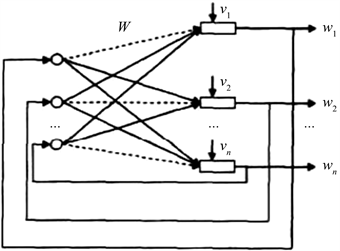Figure 7. A fully interconnected network with feedback

${E}_{i}=-\left(\underset{j\ne 1}{\overset{n}{\sum }}{w}_{ij}{v}_{i}-{\theta }_{i}\right)v$

${E}_{i}=-\frac{1}{2}\underset{i=1}{\overset{n}{\sum }}\underset{j\ne i}{\overset{n}{\sum }}\text{\hspace{0.17em}}{w}_{ij}{v}_{i}{v}_{j}+\underset{i=1}{\overset{n}{\sum }}\text{\hspace{0.17em}}{\theta }_{i}{v}_{i}$

${u}_{i}\left(t\right)={\sum }_{j\ne i}^{n}{w}_{ij}{v}_{i}-{\theta }_{i}$

if $\left({u}_{i}\left(t\right)\right)\ge 0$

then ${v}_{i}\left(t+1\right)=1$

else ${v}_{i}\left(t+1\right)=0$

Hopfield网络具固定的稳定状态，权矩阵W可以根据网络的学习求得，再利用计算的方法进行联想。对于给出的M个模式，可以使用Hebb规则进行学习。

${W}_{ij}=\left\{\begin{array}{ll}0\hfill & i=j\hfill \\ \underset{k=1}{\overset{M}{\sum }}\text{\hspace{0.17em}}{v}_{i}\left(k\right){v}_{j}\left(k\right)\hfill & i\ne j\hfill \end{array}$

3.2. Hopfield神经网络建立

3.2.1. 输入样本的设计

0~9中的每个数可以用带布尔值的7*5矩阵表示。比如“0”可以表示为：

$r0=\left[\begin{array}{ccccc}0& 0& 1& 0& 0\\ 0& 1& 1& 1& 0\\ 1& 1& 0& 1& 1\\ 1& 0& 0& 0& 1\\ 1& 1& 0& 1& 1\\ 0& 1& 1& 1& 0\\ 0& 0& 1& 0& 0\end{array}\right]$.

$\text{Number}=\left[r1,r2,r3,r4,r5,r6,r7,r8,r9,r10\right]$.

3.2.2. 输出样本的设计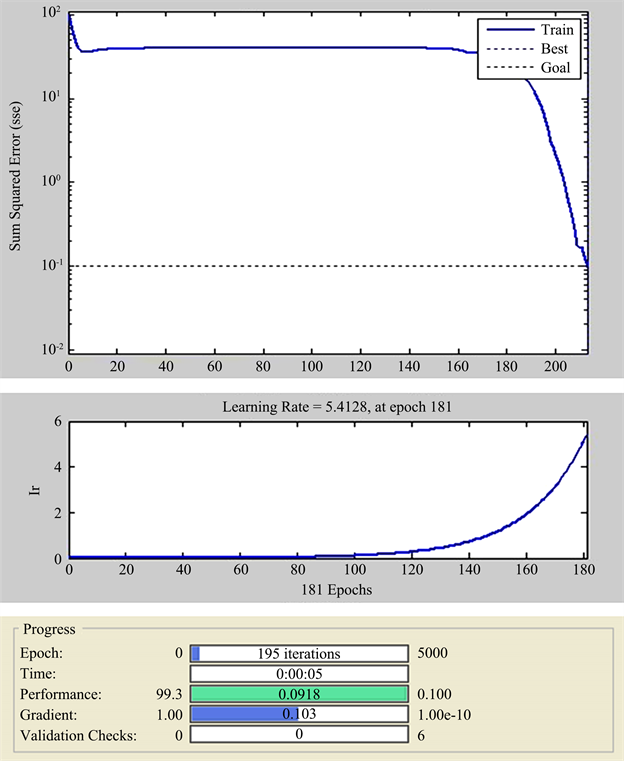Figure 8. The sum of error squares and the change in learning rate

Targets = eye (10)。

3.2.3. 网络结构的设计

3.2.4. 初始化

3.3. 无噪声网络训练

3.4. 网络测试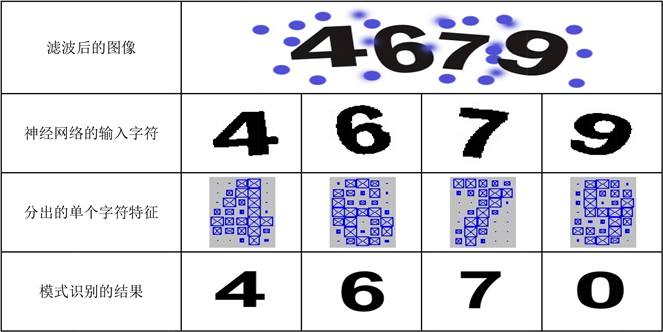Figure 9. Test result

4. 小结

NOTES

*通讯作者。

  王斌君, 王靖亚, 杜凯选, 韩宇. 验证码技术的攻防对策研究[J]. 计算机应用研究, 2013, 30(9): 2776-2779.  文晓阳, 高能, 夏鲁宁, 荆继武. 高效的验证码识别技术与验证码分类思想[J]. 计算机工程, 2009, 35(8): 186-188+191.  李建成, 李富城, 刘建芳. 基于卷积神经网络的数字验证码识别研究[J]. 电子设计工程, 2019, 27(17): 107-111.  潘浩, 李兰. 基于Tesseract引擎样本训练的验证码识别[J]. 信息与电脑(理论版), 2020, 32(1): 138-139+142.  冯军军, 王海沛, 陈新华. 基于Python3的极验验证码识别的研究[J]. 电脑知识与技术, 2019, 15(22): 37-39.  陈广秋, 王冰雪, 刘美, 刘广文. 基于结构信息相似度的线性投影灰度化算法[J]. 2020, 57(4): 877-884.  陈海峰, 丁丽丽. 二值化图像的灰度处理算法研究[J]. 电脑与电信, 2019(7): 34-38.  李治江, 丛林. 基于朴素贝叶斯理论的彩色图像二值化研究方法[J]. 数字印刷, 2020(1): 17-21.  钟彩, 杨兴耀. 车牌定位与车牌分割技术研究[J]. 电脑知识与技术, 2018, 14(2): 172-173.  张萍. 基于MATLAB的汽车牌照自动识别技术研究[J]. 自动化技术与应用, 2019, 38(11): 132-135+149.  皇甫磊磊, 阎瑞兵, 赵晓晓. 离散Hopfield神经网络在车牌识别系统中的应用[J]. 信息与电脑(理论版), 2018(17): 81-84.  徐展彧. 一种基于卷积神经网络的验证码识别方法[P]. 中国, CN110276357A. 2019-09-24.  张圣洁, 秦祎晗, 刘奕慧, 郭玮. 用于验证码识别的神经网络模型的构建方法和装置[P]. 中国, CN107360137A. 2017-11-17.  吕霁. 基于神经网络的验证码识别技术研究[D]: [硕士学位论文]. 泉州: 华侨大学, 2015.  李世成, 东野长磊. 基于卷积神经网络的验证码识别[J]. 软件, 2020, 41(4): 173-177.  杨晓帆, 陈廷槐. 人工神经网络固有的优点和缺点[J]. 计算机科学, 1994, 21(2): 23-26.